# C++ Array - Learn C++ - C++ Tutorial - C++ programming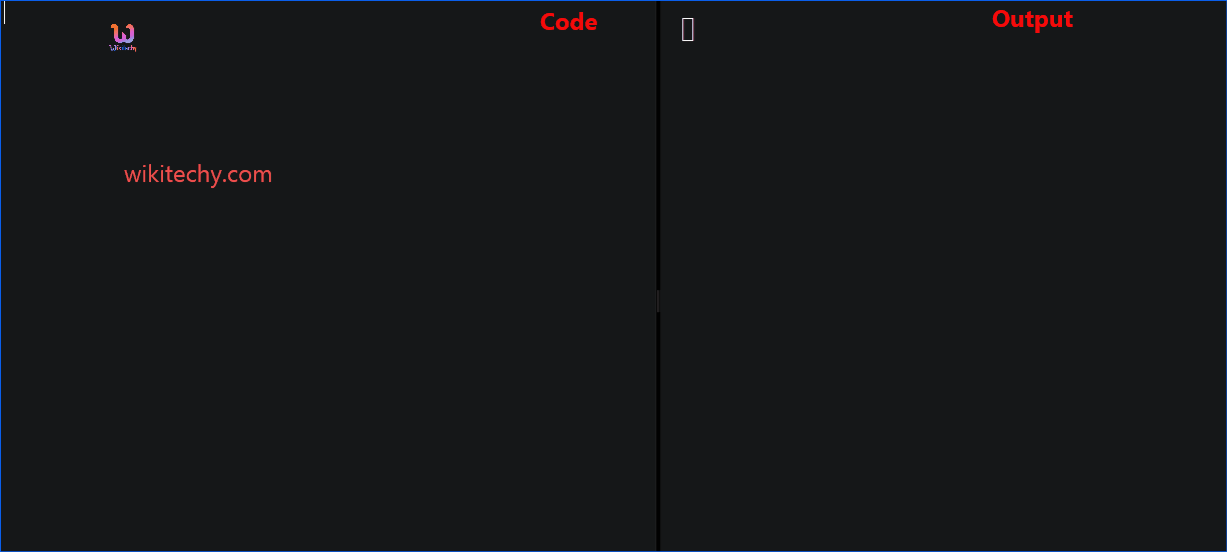Learn c++ - c++ tutorial - c++ arrays - c++ examples - c++ programs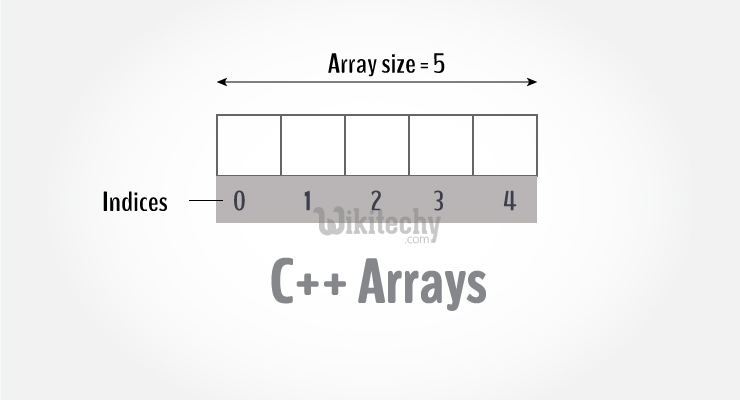## Arrays:

• C++ provides a data structure, the array, which stores a fixed-size sequential collection of elements of the same type.
• An array is used to store a collection of data, but it is often more useful to think of an array as a collection of variables of the same type.
•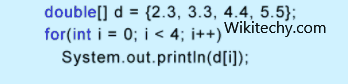learn c++ tutorials - arrays Example

## For example:

• Here, the age array can hold maximum of 100 elements of integer type.
• The size and type of arrays cannot be changed after its declaration.

## For example,

• Here, we declared an array, mark, of floating-point type and size 5. Meaning, it can hold 5 floating-point values.

## Elements of an Array and How to access them?

• You can access elements of an array by using indices.
• Suppose you declared an array mark as above. The first element is mark, second element is mark and so on.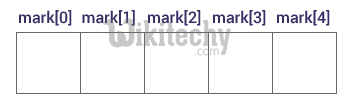## Few key notes:

• Arrays have 0 as the first index not 1. In this example, mark is the first element.
• If the size of an array is n, to access the last element, (n-1) index is used. In this example, mark is the last element.
• Suppose the starting address of mark is 2120d. Then, the next address, a, will be 2124d, address of a will be 2128d and so on. It's because the size of a float is 4 bytes.

## How to initialize an array in C++ programming?

• It's possible to initialize an array during declaration. For example,
• Another method to initialize array during declaration: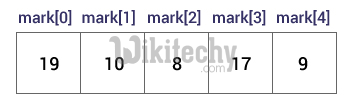• Here,

## Example: C++ Array

• C++ program to store and calculate the sum of 5 numbers entered by the user using arrays.

## Multidimensional Arrays in C++: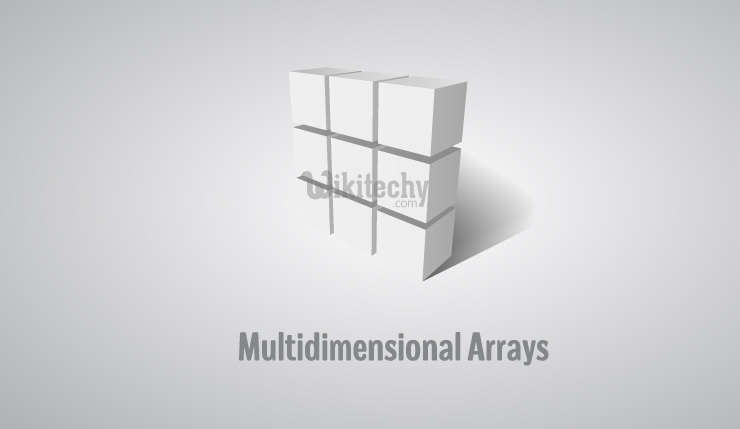• In C++, you can create an array of an array known as multi-dimensional array. For example:
• Here, x is a two dimensional array. It can hold a maximum of 12 elements.
• You can think this array as table with 3 rows and each row has 4 columns as shown below.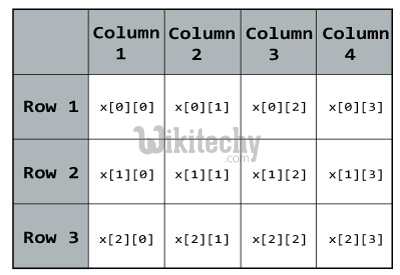• Three dimensional array also works in a similar way. For example:
• This array x can hold a maximum of 24 elements. You can think this example as: Each of the 2 elements can hold 4 elements, which makes 8 elements and each of those 8 elements can hold 3 elements. Hence, total number of elements this array can hold is 24.

## Multidimensional Array Initialization:

• You can initialize a multidimensional array in more than one way.

## Initialisation of two dimensional array

• Better way to initialize this array with same array elements as above.

## Example :Two Dimensional Array

• C++ Program to display all elements of an initialised two dimensional array.